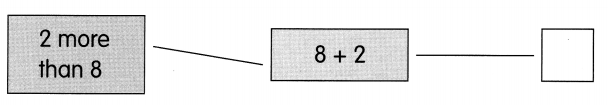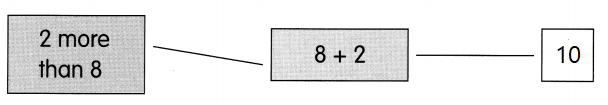This handy Math in Focus Grade 1 Workbook Answer Key Chapter 3 Practice 1 Ways to Add detailed solutions for the textbook questions.

Add. Count on from the greater number.

Example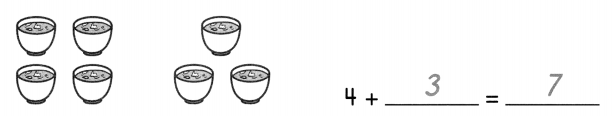Question 1.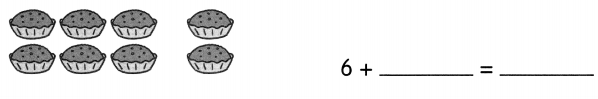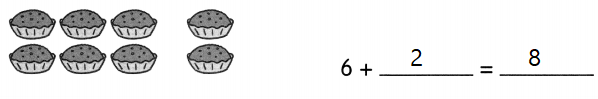Explanation:
There are 3 muffins and the chef prepared 2 more muffins
6 + 2 = 8
The sum of 6 and 2 is 8

Question 2.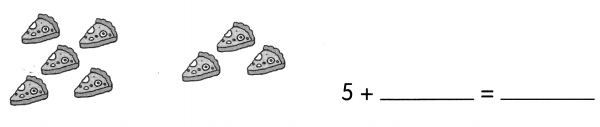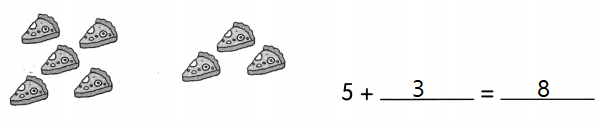Explanation:
In one pizza they made 5 pieces
and in the other pizza they made 3 pieces
5 + 3 = 8
The sum of 5 and 3 is 8

Question 3.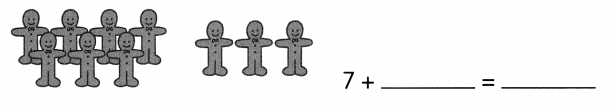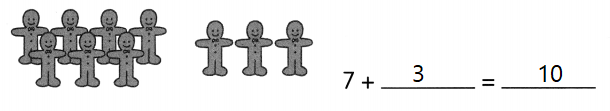Explanation:
In one page there are 7 stickers
and in the other page there are 3 stickers
7 + 3 = 10
The sum of 7 and 3 is 10

Question 4.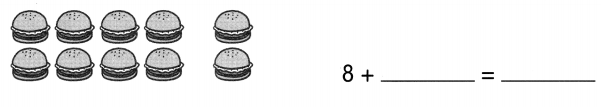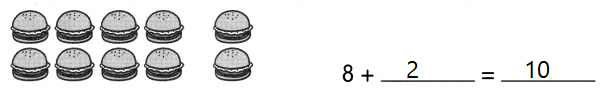Explanation:
A man ordered 8 burgers and then he added 2 more burgers
8 + 2 = 10
the sum of 8 and 2 is 10

Look at the pictures.

Add. Count on from the greater number.

Example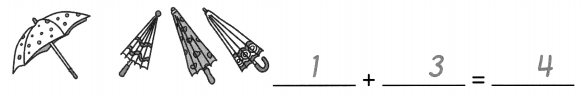Question 5.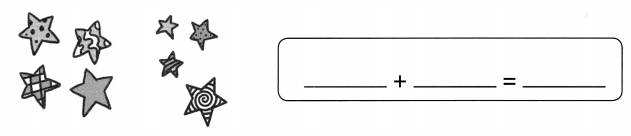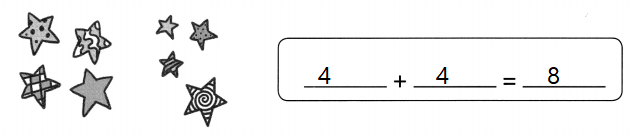Explanation:
A girl saws 4 stars and a boy saw another group of 4 stars
4 + 4 = 8
the sum 4 and 4 is 8

Question 6.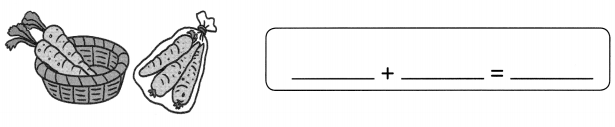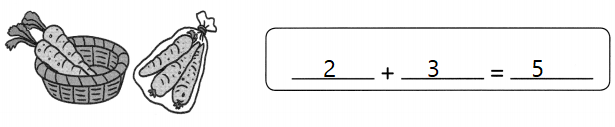Explanation:
In one basket there are 2 carrots and in the bag there are 3 carrots
2 + 3 = 5
The sum of 2 and 3 is 5

Question 7.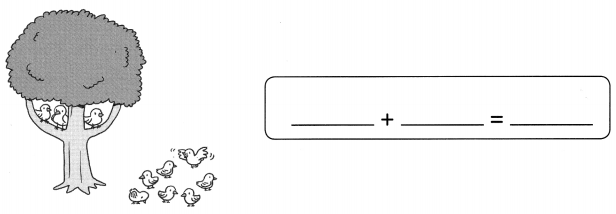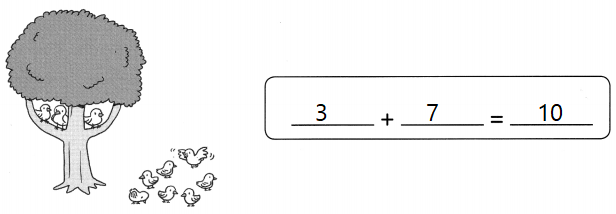Explanation:
There are 3 birds on the tree
7 birds on the ground
3 + 7 = 10
The sum of 3 and 7 is 10.

Question 8.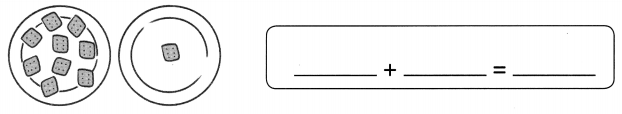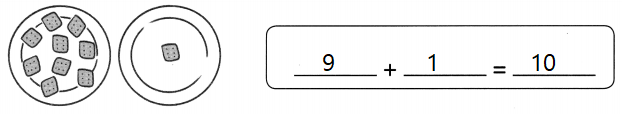Explanation:
In one plate there are 9 cookies and in other plate there is only one cookie
9 + 1 = 10
The sum of 9 and 1 is 10.

Question 9.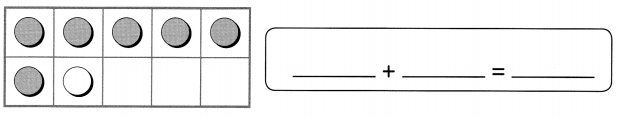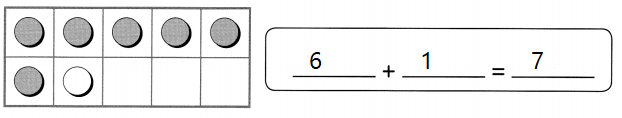Explanation:
In a ten frame there are 6 colored counters
and 1 non colored counter
6 + 1 = 7
The sum of 6 and 1 is 7

Question 10.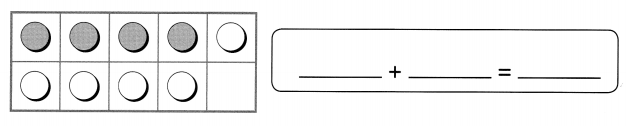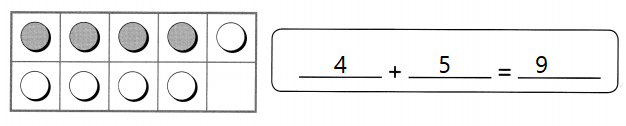Explanation:
In a ten frame there are 4 colored counters
and 5 non colored counter
4 + 5 = 9
The sum of 4 and 5 is 9

Question 11.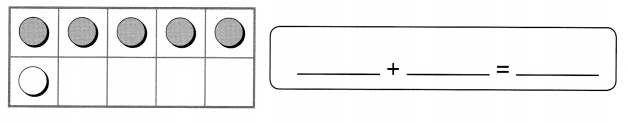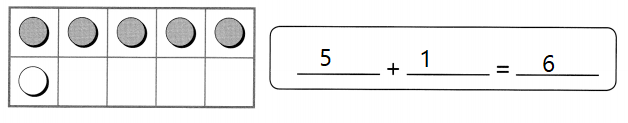Explanation:
In a ten frame there are 5 colored counters
and 1 non colored counter
5 + 1 = 6
The sum of 5 and 1 is 6

Question 12.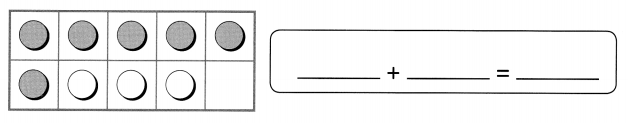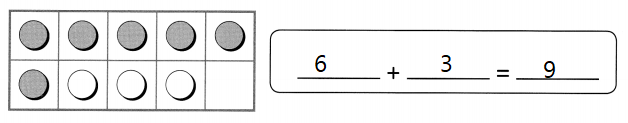Explanation:
In a ten frame there are 6 colored counters
and 3 non colored counter
6 + 3 = 9
The sum of 6 and 3 is 9

Count on from the greater number to add.

Question 13.
4 + 1 = ___
5
Explanation:
The sum of 4 and 1 is 5
4 + 1 = 5

Question 14.
6 + 2 = ______
8
Explanation:
the sum of 6 and 2 is 8
6 + 2 = 8

Question 15.
9 + 1 = ___
10
Explanation:
The sum of 9 and 1 is 10
9 + 1 = 10

Question 16.
3 + 4 = ______
7
Explanation:
The sum of 3 and 4 is 7
3 + 4 = 7

Question 17.
3 + 7 = ______
10
Explanation:
The sum of 3 and 7 is 10
3 + 7 = 10

Question 18.
4 + 5 = ______
9
Explanation:
The sum of 4 and 5 is 9
4 + 5 = 9

Complete.
Write the answer in each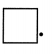Example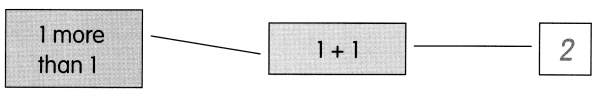Question 19.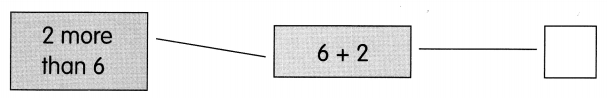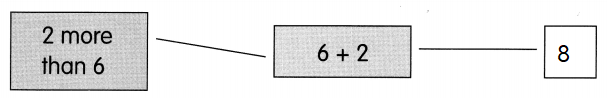Explanation:
2 more than 6 is 8
so, we have to add 2 to 6
6 + 2 = 8

Question 20.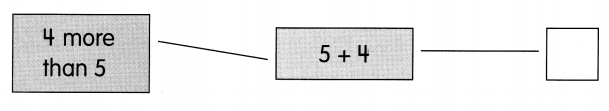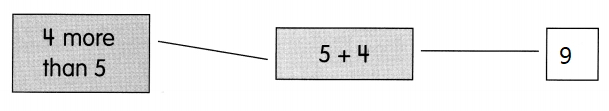Explanation:
4 more than 5 is 9
so, we have to add 4 to the 5
4 + 5 = 9

Question 21.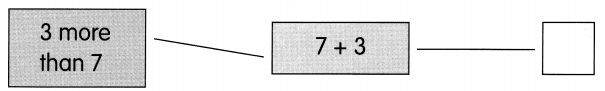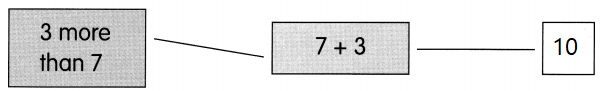Explanation:
3 more than 7 is 10
so, we have to add 3 to the number 7
3 + 7 = 10

Question 22.